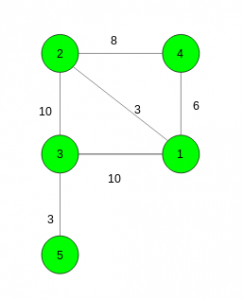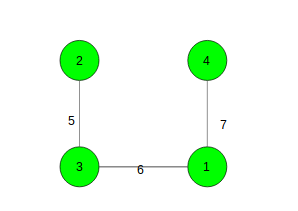Shortest path from source to destination such that edge weights along path are alternatively increasing and decreasing

• Difficulty Level : Hard
• Last Updated : 24 Apr, 2019

Given a connected graph with N vertices and M edges. The task is to find the shortest path from source to the destination vertex such that the difference between adjacent edge weights in the shortest path change from positive to negative and vice versa ( Weight(E1) > Weight(E2) < Weight(E3) …. ). If no such path exists then print -1.

Examples:

Input: source = 4, destination = 3Output: 19
4 – 2 – 1 – 3 (Edge Weights: 8, 3, 10) and 4 – 1 – 2 – 3 (Edge Weights: 6, 3, 10) are the only valid paths.
Second path takes the minimum cost i.e. 19.

Input: source = 2, destination = 4Output: -1
No such path exists.

Recommended: Please try your approach on {IDE} first, before moving on to the solution.

Approach: Here, We need to keep two copies of adjacent lists one for positive difference and other for negative difference. Take a Priority Queue as in Dijkstras Algorithm and keep four variables at a time i.e.,

1. cost: To store the cost of the path till current node.
2. stage: An integer variable to tell what element needs to be taken next, if the previous value was negative then a positive value needs to be taken else take negative.
3. weight: Weight of the last visited node.
4. vertex: Last visited vertex.

For every vertex push the adjacent vertices based on the required condition (value of stage). See the code for better understanding.

Below is the implementation of the above approach:

 // C++ implementation of the approach #include using namespace std; #define N 100005    // To store the graph vector > incr[N], decr[N]; int _incr[N], _decr[N], shortest[N];    int n, m, src, dest, MAXI = 1LL << 30;    // Function to add edges void Add_edge(int x, int y, int w) {     incr[x].push_back({ w, y });     incr[y].push_back({ w, x });     decr[x].push_back({ -w, y });     decr[y].push_back({ -w, x }); }    // Function to find the shortest distance from // source to destination int Modified_Dijkstra() {        // Total cost, stage, weight of previous, vertex     priority_queue, pair > > q;        // Sort the edges     for (int i = 1; i <= n; i++) {         sort(incr[i].begin(), incr[i].end());         sort(decr[i].begin(), decr[i].end());     }        for (int i = 1; i <= n; i++)         shortest[i] = MAXI;        // Push the source vertex     q.push({ { 0, 0 }, { 0, src } });        while (!q.empty()) {            // Take the top element in the queue         pair, pair > FRONT = q.top();            // Remove it from the queue         q.pop();            // Store all the values         int cost = -FRONT.first.first;         int stage = FRONT.first.second;         int weight = FRONT.second.first;         int v = FRONT.second.second;            // Take the minimum cost for the vertex         shortest[v] = min(shortest[v], cost);            // If destination vertex has already been visited         if (shortest[dest] != MAXI)             break;            // To make difference negative         if (stage) {                // Start from last not visited vertex             for (int i = _incr[v]; i < incr[v].size(); i++) {                    // If we can take the ith vertex                 if (weight > incr[v][i].first)                     q.push({ { -(cost + incr[v][i].first), 0 },                              { incr[v][i].first, incr[v][i].second } });                 else {                        // To keep the last not visited vertex                     _incr[v] = i;                     break;                 }             }         }            // To make difference positive         else {                // Start from last not visited vertex             for (int i = _decr[v]; i < decr[v].size(); i++) {                    // If we can take the ith vertex                 if (weight < -decr[v][i].first)                     q.push({ { -(cost - decr[v][i].first), 1 },                              { -decr[v][i].first, decr[v][i].second } });                 else {                        // To keep the last not visited vertex                     _decr[v] = i;                     break;                 }             }         }     }        if (shortest[dest] == MAXI)         return -1;        return shortest[dest]; }    // Driver code int main() {     n = 5, src = 4, dest = 3;        // Adding edges     Add_edge(4, 2, 8);     Add_edge(1, 4, 6);     Add_edge(2, 3, 10);     Add_edge(3, 1, 10);     Add_edge(1, 2, 3);     Add_edge(3, 5, 3);        cout << Modified_Dijkstra();        return 0; }

Output:

19

My Personal Notes arrow_drop_up
Recommended Articles
Page :